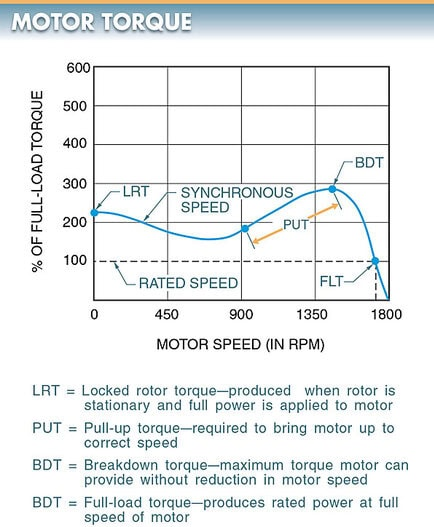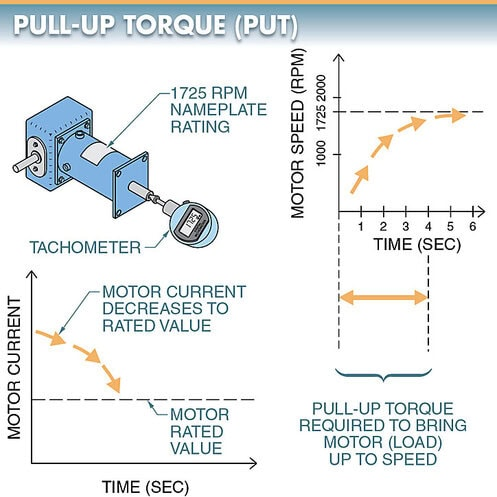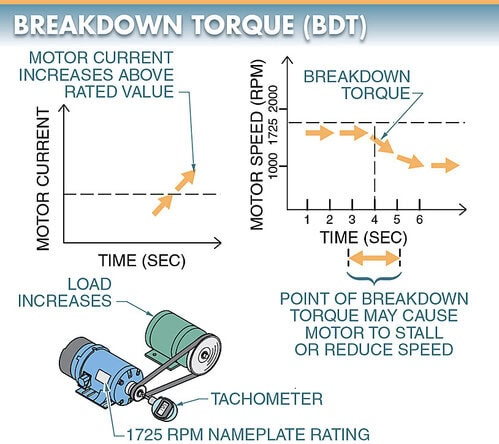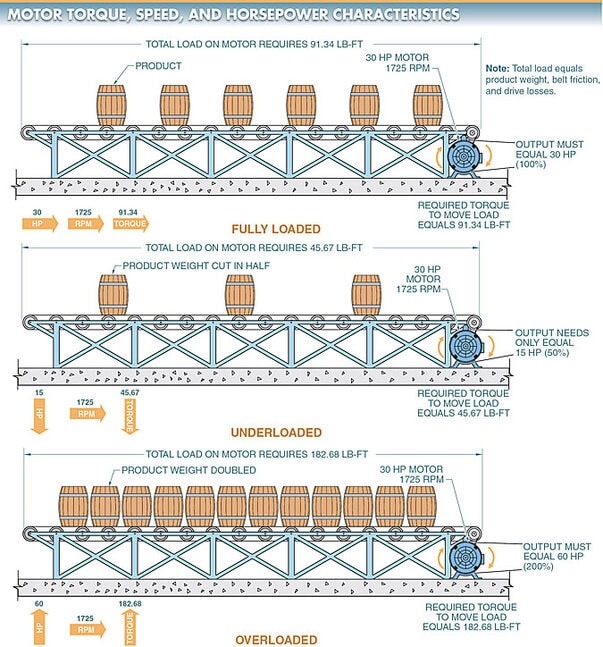# Electric Motor Torque Types | Locked Rotor Torque, Pull-Up Torque, Breakdown Torque, Full-Load Torque

Want create site? Find Free WordPress Themes and plugins.

An electric motor must produce enough torque to start the load and keep it moving for the motor to operate the load connected to it. A motor connected to a load produces four types of torque. The four types of torque are locked rotor torque (LRT), pull-up torque (PUT), breakdown torque (BDT), and full-load torque (FLT). See Figure 1.Figure 1. A motor connected to a load produces four types of torque: locked rotor torque (LRT), pull-up torque (PUT), breakdown torque (BDT), and full-load torque (FLT).

## Locked Rotor Torque

Locked rotor torque (LRT) is the torque a motor produces when its rotor is stationary and full power is applied to the motor. See Figure 2.

All motors can safely produce a higher torque output than the rated full-load torque for short periods of time. Since many loads require a higher torque to start them moving than to keep them moving, a motor must produce a higher torque when starting the load.

LRT is also referred to as breakaway or starting torque. Starting torque is the torque required to start a motor and is normally expressed as a percentage of full-load torque.Figure 2. Locked rotor torque (LRT) is the torque a motor produces when its rotor is stationary and full power is applied to the motor.

## Pull-Up Torque

Pull-up torque (PUT) is the torque required to bring a load up to its rated speed. See Figure 3. If a motor is properly sized to the load, PUT is brief.

If a motor does not have sufficient PUT, the locked rotor torque may start the load turning but the PUT cannot bring it up to rated speed. Once the motor is up to rated speed, full-load torque keeps the load turning. PUT is also referred to as accelerating torque.Figure 3. Pull-up torque (PUT) is the torque required to bring a load up to its rated speed.

Breakdown Torque

Breakdown torque (BDT) is the maximum torque a motor can provide without an abrupt reduction in motor speed. See Figure 4.

As the load on a motor shaft increases, the motor produces more torque. As the load continues to increase, the point at which the motor stalls is reached. This point is the breakdown torque.Figure 4. Breakdown torque (BDT) is the maximum torque a motor can provide without an abrupt reduction in motor speed.

Full-load torque (FLT) is the torque required to produce the rated power at the full speed of the motor. See Figure 5.

The amount of torque a motor produces at rated power and full speed (full-load torque) can be found by using a horsepower-to-torque conversion chart.Figure 5. Full-load torque (FLT) is the torque required to produce the rated power at full speed of the motor.

To calculate motor FLT, the following formula is applied:

$T=\frac{HP\times 5252}{RPM}$

Where

T = torque (in lb-ft)

HP = horsepower 5252 = constant

RPM = revolutions per minute

What is the FLT of a 30 HP motor operating at 1725 rpm?

Solution

$T=\frac{HP\times 5252}{RPM}=\frac{30\times 5252}{1725}=91.34lb-ft$

If a motor is fully loaded, it produces FLT. If a motor is underloaded, it produces less than FLT. If a motor is overloaded, it must produce more than FLT to keep the load operating at the motor’s rated speed. See Figure 6.